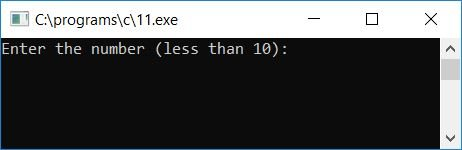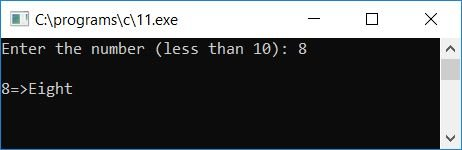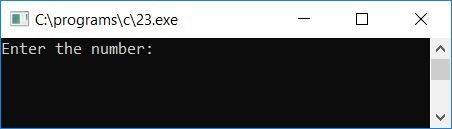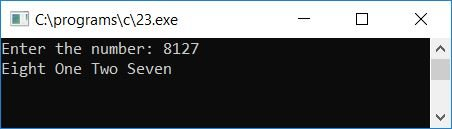# C program to print the given number in words

In this article, we will learn how to create a program in C that will ask the user to enter any number to convert that number into words (characters).

## Print a single-digit number in Word

Let's first create a program that will print any given one-digit number (provided by the user at run-time) in words. For example, if the user enters 7, then the program prints "Seven" as output. Later on, you will be given the code for printing a number (with more than one digit) in words.

```#include<stdio.h>
#include<conio.h>
int main()
{
int num;
printf("Enter the number (less than 10): ");
scanf("%d", &num);
printf("\n");
if(num<10 && num>0)
{
if(num==1)
printf("1=>One");
else if(num==2)
printf("2=>Two");
else if(num==3)
printf("3=>Three");
else if(num==4)
printf("4=>Four");
else if(num==5)
printf("5=>Five");
else if(num==6)
printf("6=>Six");
else if(num==7)
printf("7=>Seven");
else if(num==8)
printf("8=>Eight");
else if(num==9)
printf("9=>Nine");
}
else
printf("Number must be in between 0 and 10");
getch();
return 0;
}```

As the program was written in the Code::Blocks IDE, here is the sample run after a successful build and run:Let's supply any number under 10, say 8, and press the ENTER key to see the output:#### Program Explained

• Receive any number as input.
• Use an if statement to check whether the given number is greater than 0 and less than 10 or not.
• If it is, then process the next steps to print the number in words; otherwise, exit from the program after printing some message like, "number must be in between 0 and 10."
• I added an if-else statement inside the if block to check the number entered by the user; if it is 1, print it out as 1=>One, if it is 2, print it out as 2=>Two,..., if it is 9, print it out as 9=>Nine.

## Print a Multiple-Digit Number in Words

Let's create another program that prints any number (more than one digit) by its digit-by-digit name (series of words). For example, if the user enters 8127, then the program prints its output as "Eight One Two Seven."

```#include<stdio.h>
#include<conio.h>
int main()
{
long int num, rev=0;
int digit, rem;
printf("Enter the number: ");
scanf("%ld", &num);
while(num>0)
{
rem = num%10;
rev = rev*10 + rem;
num = num/10;
}
while(rev>0)
{
digit = rev%10;
switch(digit)
{
case 1: printf("One ");
break;
case 2: printf("Two ");
break;
case 3: printf("Three ");
break;
case 4: printf("Four ");
break;
case 5: printf("Five ");
break;
case 6: printf("Six ");
break;
case 7: printf("Seven ");
break;
case 8: printf("Eight ");
break;
case 9: printf("Nine ");
break;
case 0: printf("Zero ");
break;
default: printf("Something went wrong!!");
break;
}
rev = rev/10;
}
getch();
return 0;
}```

Here is the sample run after a successful build and run:Now supply any number, say 8127, as input and press the ENTER key. Here is the output you will see:#### Program Explained

• Receive any number as input from the user at run-time.
• Here we have reversed the number first, before printing it by its digit-by-digit name.
• Because whenever we use the modulus (%) operator to find the remainder or digit of the number, we will always get the last digit of the number.
• For example, if the user enters 8127 as input, then the first time the remainder or modulus operator is applied to the number 8127, that is rem = num%10, where num holds 8127. That is, 8127%10 or 7 (the last digit of the number) will be initialized to "rem."
• Therefore, if we reverse the number, it becomes 7218, and now when we calculate its remainder or digit using the modulus operator, we will get 8 as its first remainder, which is the first digit of the number. Therefore, we have done the reverse operation on the given number before actually processing it to print it by its digit-by-digit name.
• To get to the point, rev is the variable that holds the number in reverse order after reversing it.
• Now find its digit using the remainder operator, that is, initialize rev%10 or 7218%10 or 8 to a variable, say digit.
• Use the switch case to determine whether the variable digit has a current value of 1 or 2 or 3... or 9 or 0.
• Print its corresponding word, and at last divide the revision number 7218 by 10 to remove its last digit, and process the same steps as told above.
• In this way, the number gets printed by its digit-by-digit name on the output screen.

C Quiz

« Previous Program Next Program »